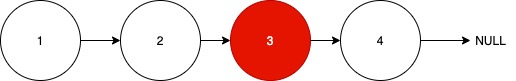Posted: 8 Dec, 2020
Difficulty: Easy

## PROBLEM STATEMENT

#### For example, let the linked list be 1->2->3->4->null#### Since the number of elements in this linked list is 4 so we have 2 middle elements, i.e. 2 and 3, but we return 3 as it is farther from the head node, i.e. 1.

##### Input Format :
``````The first line of input contains an integer ‘T’ denoting the number of test cases.

The next ‘2*T’ lines represent the ‘T’ test cases.

The first and only line of each test case contains integers denoting the nodes of the linked list. Each line is guaranteed to have -1 at the end to signify the end of the linked list.
``````
##### Output Format :
``````For each test case, return a pointer pointing to the node which is at the middle of the linked list. If no midpoint exists, return a null pointer.
``````
##### Note :
``````1.You do not need to print anything, it has already been taken care of. Just implement the given function.

2.For a linked list of size 1, the head node is the midpoint.

3.If no midpoint exists, return a null pointer.
``````
##### Constraints :
``````1 <= T <= 50
0 <= N <= 4*10^4
-10^9 <= data <= 10^9
data ≠ -1

Where 'N' is the number of nodes and 'data' is the value of nodes.

Time Limit: 1 sec
``````Approach 1
• If we want to know the midpoint of any linked list, it is very easy to do so if we know the number of elements in the linked list.
• We take a pointer ‘p’ and use it to traverse the linked list and until we find NULL we increment a variable ‘count’ to count the number of elements in the linked list.
• We then divide the number of elements by 2 to get the position of the middle element of the linked list.
• Finally, we traverse through the first n/2 elements of the list and return the pointer of the middle element of the linked list.

We can take the Following Approach:

• Take a pointer ‘p’ to traverse the linked list, initially pointing to head node.
• Take a variable ‘numberOfNodes’ to count the number of nodes in the linked list.
• Take a variable ‘mid’ and initialize it with ‘numberOfNodes/2’(middle of Linked List).
• Finally, take a pointer ‘ptr’ and traverse through ‘mid’ number of nodes.
• Return ‘ptr’ which is now at the middle of the linked list.